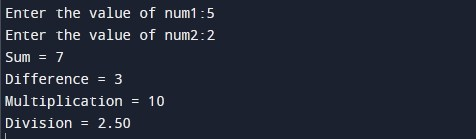# C program to perform addition, subtraction, multiplication and division

In this tutorial you will learn about the C program to perform addition, subtraction, multiplication and division and its application with practical example.

In this tutorial, we will learn to create a program to perform addition, subtraction, multiplication and division of two numbers using C programming language.

Prerequisites

Before starting with this tutorial we assume that you are best aware of the following C programming topics:

• C Data Types
• C Variables
• C Input Output
• C Operators
• C Typecasting

## Program to perform basic arithmetic operations

In this program we will accept two operands from the user. Then, we will perform the basic arithmetic operations(addition, subtraction, multiplication and division) on the two operands. Later in the program we will display the result.

Output:-In the above program, we have first declared and initialized a set variables required in the program.

• num1 = it holds the first number value
• num2 = it holds the second number value
• add = it holds the sum of two numbers
• subtract = it holds subtraction result of two numbers
• multiply = it holds the multiplication result of two numbers
• divide = it holds the division result of two numbers

In the next statement user will be prompted to enter the two number values which will be assigned to variable ‘num1’ and ‘num2’ respectively. Then, we will perform the basic arithmetic operations(addition, subtraction, multiplication and division) on the two operands and assign the result to respective variable add, subtract, multiply and divide. Finally, we will be displaying the result using printf statement.

In this tutorial we have learn about the C program to perform addition, subtraction, multiplication and division and its application with practical example. I hope you will like this tutorial.